# Electronics and Communication Engineering - Exam Questions Papers

16.

In the following circuit, the switch S is closed at t = 0 The rate of change of current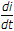(0 +) is given by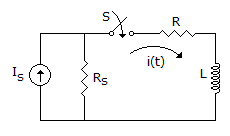A. 0 B.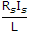C.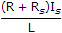D. ∞

Answer: Option B

Explanation:

At t = 0, inductor acts like a open circuit.

We know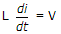at t = 0+, inductor path is open circuit

V = Is.Rs (at t = 0+)

Thus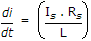17.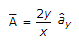convert into spherical co-ordinates.

 A. A = 2 sinθ[sinθ tanΦa r + sinΦa θ + a Φ] B. A = 2 sinΦ[sinΦ tanΦa r + sinΦa θ + a Φ] C. A = 2 sinΦ[sinΦ tanΦa r - sinΦa θ - a Φ] D. none of these

Answer: Option D

Explanation:

Spherical :

Ar = A. a r =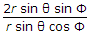.sinθ.sinφ =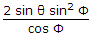Aθ = A. a θ =.cosφsinφ

= 2 sin2φ

Aφ = Aa φ =cosφ = 2 sinφ

A =a r + 2sin2φa θ + 2sinφaφ

A = 2sinφ[sinθ tanφ a r + sinφa θ + aφ].

18.

Consider the following program segment written for 8085 based system
`LXI SP, FFFFHMVI A, FFHMOV BB, AK: DCR BPUSH HLJNZ KINR ALXI H, FFCEHCPI 20HLHLD`
The contents of accumulator is :

 A. CE B. 20 C. indeterminate D. none of these

Answer: Option C

Explanation:

Since contents of HL register pair are unknown and are continuously ''PUSH''ed on the stack; retrieval of the same will result in ''Indeterminate'' data.

19.

The reduced form of the function
Y(A, B, C, D, E, F) = ∑m(0, 1, 2, 3, 4, 6, 7, 8, 9, 12, 14, 15, 16, 17, 18, 19, 20, 22, 23, 24, 25, 28, 30, 31) is given by:

 A. A(C D + E F + D E) + D E B. (A + C + D + E)(D + E + F) C. (C + D + E + A)(A + D + E + F) D. A C D + A E F + A(D ⊕ E)

Answer: Option C

Explanation: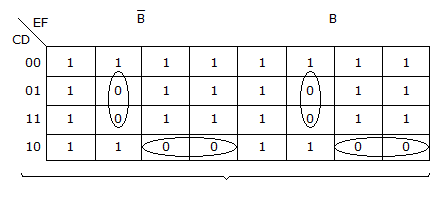Y = (A + D + E + F)(A + C + D + E).

20.

A 1000 kHz carrier wave modulated 40% at 4000 Hz is applied to a resonant circuit tuned to a carrier frequency and having Q = 140. What is the degree of modulation after transmission through this circuit?

 A. 0.4 B. 0.2 C. 0.27 D. 0.54

Answer: Option C

Explanation:

Resulting depth of modulation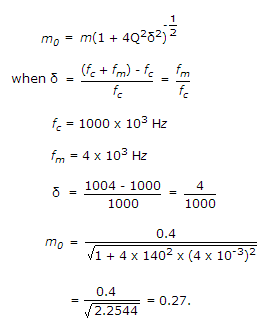#### Current Affairs 2021

Interview Questions and Answers